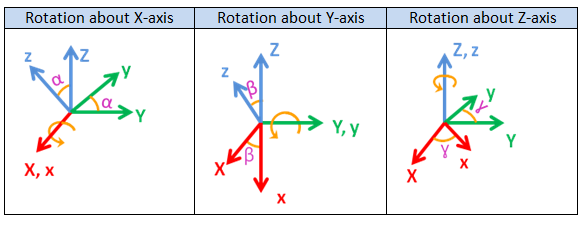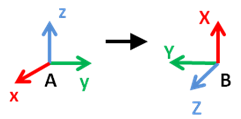top of page

Rotation Matrices

A 3D rotation matrix is of size is 3X3 and is given as below,I will clear your doubts on rotation matrices using below example.

Lets assume we have two frames A and B. Frame A is denoted by x,y,z axes and frame B is denoted by X,Y,Z axes.Fig. 1 Frame A and Frame B

To get the rotation of frame B w.r.t frame A we have to find the unit vectors [X,Y ,Z] of X,Y,Z coordinate axes in terms of [x,y,z] unit vectors of x,y,z coordinate axes.

The unit vectors [X,Y,Z] of frame B can be written in terms of unit vectors [x,y,z] of frame A as given belowSo as you see in the above equation , R11,R12,.......R33 are the 9 variables that describe the orientation of frame B w.r.t frame A.

Hence these 9 variable can be grouped into 3X3 matrix, where each column represent the target frame unit vector [X,Y,Z] .To find R11,R12,.......R33 we have to super impose frame B on frame A. I have split the diagrams for each  unit vector of frame BFig. 2 Representing Frame B unit vectors in terms of Frame A unit vectors

Coming to the calculation of R11,R12,......R33, you need to superimpose two frames and find them as shown in fig 2.

The elemental rotations along x-axis, y-axis and z-axis by angle α, β and ɣ respectively are given as belowHope now you are clear with rotation matrices and its elements.

You can find the rotation matrix intuitively by seeing the source frame and target frame

I will explain you how, with some examples.

Rotatoin Matrices intutively

1).2).Now its your turn to find the below rotation matrices.